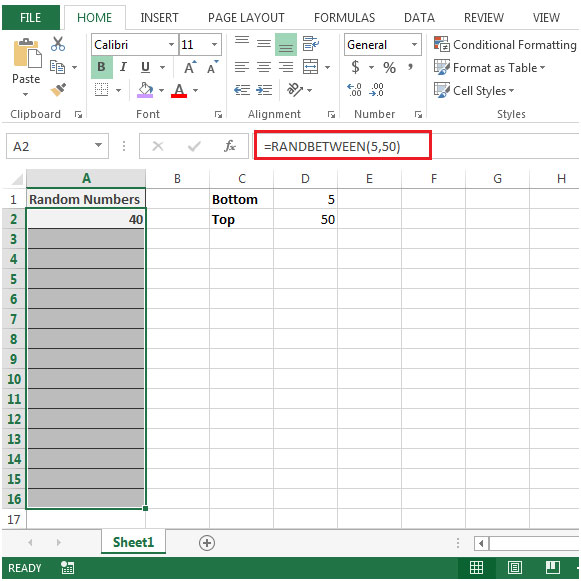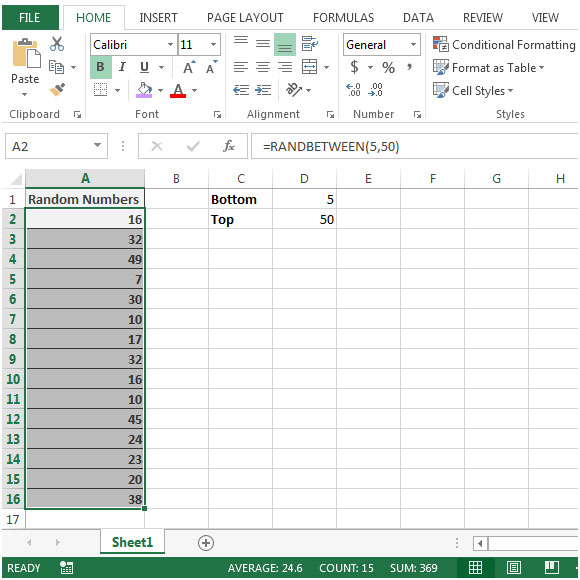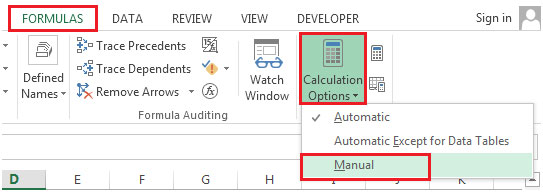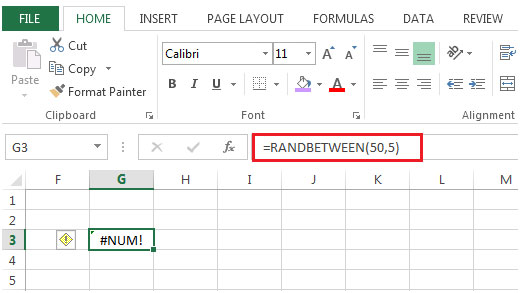# Generate a Random Value between Two Limits in Microsoft Excel

In this article, we will learn how to generate random number between two values in Microsoft Excel.

To generate a list of the random values between two limits, we will use of RANDBETWEEN function.

Example of RANDBETWEEN function used in our life is ATM PIN, Net Banking password.

RANDBETWEEN returns a random integer number between the two specified (bottom & top) numbers. A new random integer number gets generated every time the worksheet is calculated.

Syntax: =RANDBETWEEN(bottom,top)

bottom: It is the smallest integer value that RANDBETWEEN will return.

top: It is the largest integer value that RANDBETWEEN will return.

Note: If bottom value is greater than top value then RANDBETWEEN function will return #NUM! error.

Let us take an example:

• You want to generate a list of random numbers in column A
• The two arguments i.e. bottom & top have assigned with the values as 5 and 50
• Select the cell in which you want to generate random number list.
• Enter the formula in range =RANDBETWEEN(5,50)• Copy the formula down in below range of cells• You will notice in cell A2, the number gets automatically changed from 40 to 16.
• This is because of the automatic calculation feature in Excel. If you do not want Excel to generate a new list or disable to re-calculate feature.
• You need to click on Formulas ribbon
• In Calculation group, click on Calculation Options• Select Manual

If you enter bottom value greater than top value then RANDBETWEEN function will return #NUM! error.

Formula in cell G3 =RANDBETWEEN(50,5) will return #NUM! error.In this way we can generate Random numbers using Excel Randbetween function.

## Users are saying about us...

1."this formula does not work in my Excel 95.
actually, what I'm looking for is a ""formula"" that will generate, for example, 24 non repeating numbers between 1 and 24."

2."1- install Analysys ToolPak
2-in cell A1 enter a bottom number, in cell a2 enter the top number"

Terms and Conditions of use

The applications/code on this site are distributed as is and without warranties or liability. In no event shall the owner of the copyrights, or the authors of the applications/code be liable for any loss of profit, any problems or any damage resulting from the use or evaluation of the applications/code.